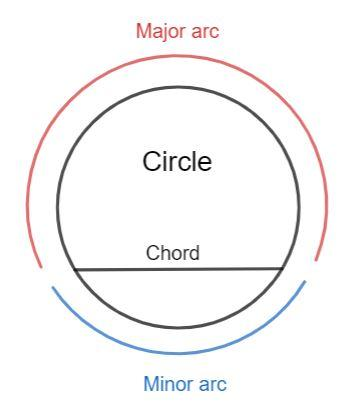QUESTION

# What is the line segment joining any two points on a circle called?(a) arc of the chord(b) radius of the circle(c) chord of the circle(d) tangent of the circle

Hint: Recall the definitions of the arc of the chord, radius of the circle, chord of the circle, and tangent of the circle. A line segment joining any two points on a conic section is called a chord.

Complete Step-by-Step solution:
A circle is a round-shaped figure having no corners or edges. It is a closed figure.
The center of a circle is defined as the fixed point inside the circle from which all the points on the circle are at equal distance.
The distance from the center of the circle to any point on the circle is called the radius of the circle.
A circle is completely represented by its center point and the radius of the circle.
The tangent to a circle is a straight line that touches a circle only at one point. The tangent of the circle is perpendicular to the radius of the circle at the point of contact.A chord of the circle is the line segment joining any two points on the circle. The part of the circumference subtended by the chord is called the arc of the chord. A chord divided the circle into the minor arc and the major arc. The longest chord is the diameter of the circle.
Hence, the line segment joining any two points on a circle is called the chord.
Hence, the correct answer is option (c).

Note: You might choose the arc of the chord as the correct answer, but the arc of a chord is a curve and not a straight line. The chord is a straight line.